# PZT Disc in Load in Analyst

## Introduction

We will look into setting up a simple PZT 2D Disc example residing in a water load.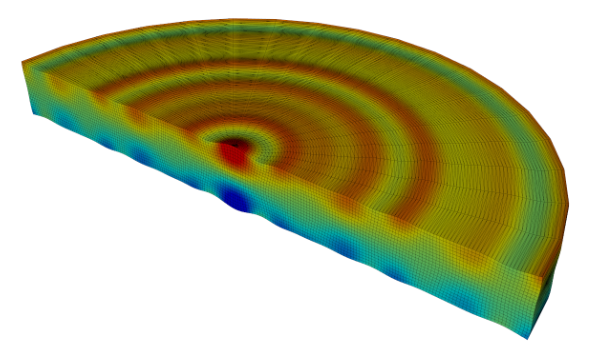### Model Inputs

• Default commands
• Parameterisation

### Meshing

• Mesh Settings
• Keypoint Generation and Grid Setup

### Materials

• Material Properties
• Assigning to Grid

• Boundaries of the Model
• Time Functions
• Electrical Conditions

### Model Outputs

• Calculated Properties
• Output Types

• PRCS Command
• Model Check

### Model Execution

• Execution Setup
• Plot Settings
• Methods of Running a Model
• Extracting Outputs

## Creating a Model Folder

Before we start to code, we will need to set up a folder to hold the files we will be using the new file explorer:

1. Open OnScale in Analyst Mode
2. Create a new folder and rename it PZT Disc Example in a directory of your choosing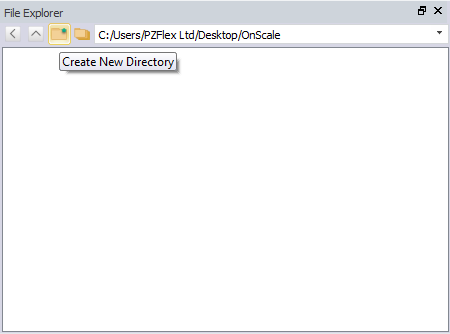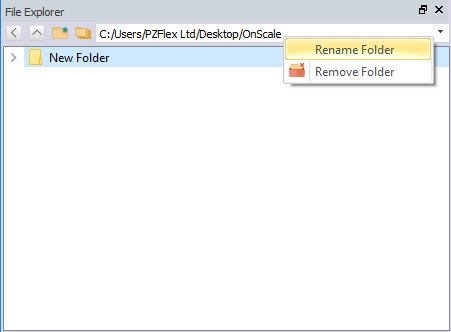1. Navigate into the folder by double clicking the folder
2. Click save (or CTRL+S) and navigate to the PZT Disc Example folder
3. Double check file is save as .flxinp
4. Name the file PZT2D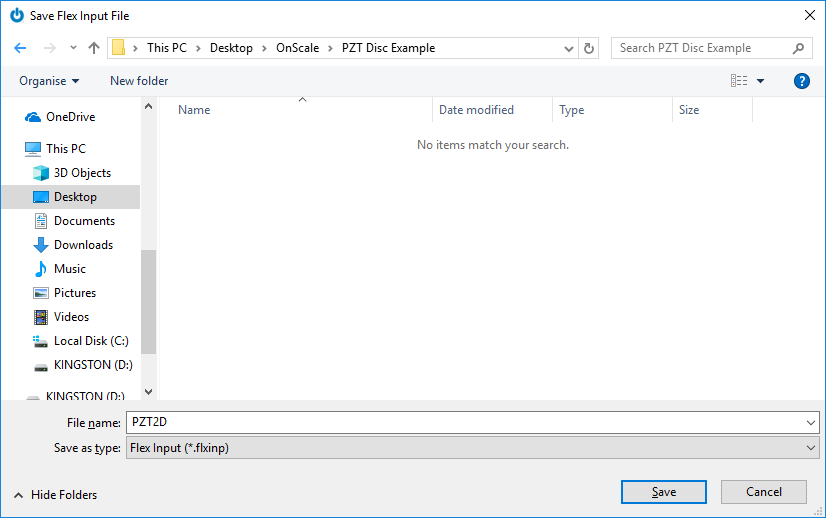7. Save file

The file explorer should now contain the file PZT2D.flxinp

You are now ready to code!!

## Model Inputs

### Default Commands

The first lines of code in a model file should typically start with comments with basic information such as:

• Author of the Model File
• Description of the model
• Date
• Version Number
• Any other useful information worth sharing to another OnScale use
`cc designer: onscalec model description: pzt 2d axisymmetric discc date: dd/mm/yyyyc version: 1.0c`

There are 3 lines of commands that usually follow:

• titl - Provide titling information and identifiers for output results
• mp omp - Provide titling information and identifiers for output results
• rest - Provide titling information and identifiers for output results
```c set model title
titl training pzt 2d disc examplec use all cores
mp omp  * *
c do not save restart file
rest no```

### Parameterization

Parameterizing the model allows changes to applied to your model very quickly and intuitively. We should always be using variables where possible to make setting up the model a simple as possible. For our PZT 2D disc example, we must identify critical dimensions that can be used to fully define the geometry - it is same process a mechanical engineer would go through to draft up a mechanical drawing of a component.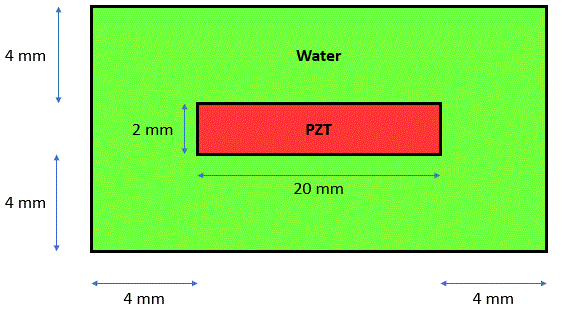Tip: Always use symmetry to reduce model size and simplify the model

As the model will be axisymmetric around the Y axis, we can apply symmetry vertically through the centre of the geometry: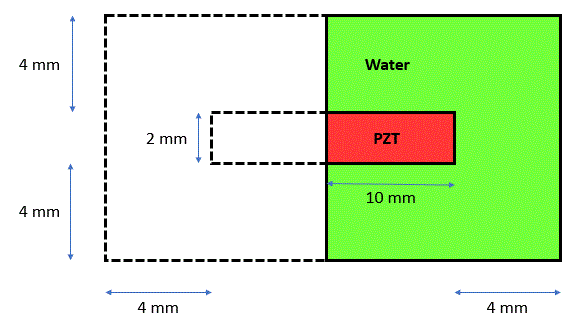Note: Although the geometry is quarter symmetric, we cannot apply horizontal symmetry as the electrical loading conditions will not be symmetric across the X-axis. Always be aware of how symmetry will affect other aspects of your model.

3 variables are all that is required to fully define this geometry:

`c geometrysymb water_size = 4e-3/* dimension of water around transducer symb pzt_rad = 10e-3    /* pzt radiussymb pzt_thk = 2e-3/* pzt thickness`

Tip: For more complex model there will be many more variables so always try to give meaningful variable names
Always maintain consistent units of measurement throughout the model - OnScale typically operates in SI units.

### Model Input Section Code

`cc designer: onscalec model description: pzt 2d axisymmetric discc date: dd/mm/yyyyc version: 1.0cc set model titletitl training pzt 2d disc examplec use all coresmp  omp  * * c do not save restart file rest  noc geometrysymb water_size = 4e-3 /* dimension of water around transducer symb pzt_rad = 10e-3   /* pzt radiussymb pzt_thk = 2e-3    /* pzt thickness`

## Meshing

### Mesh Settings

Meshing is one of the key components to obtaining accurate results. The elements that are used to construct a mesh must take into account a number of aspects to be able to discretise stress gradients accurately within the model. The following parameters are typically used to determine the required element size which we commonly refer to as box:

1. Frequency of interest
• Typical variable name - freqint
• Set to the centre of the frequency band that you are interested in
2. Slowest wave velocity in the model
• Typical Variable name - vel
• Generally set to the lowest shear wave velocity in the model
3. Shortest wavelength in the model
• Typical variable name - wavelength
• Calculation of the shortest wavelength based on vel and freqint
4. Number of elements to discretise a wavelength
• Typical variable name - nepw
• Minimum value of 15 elements per wavelength is recommended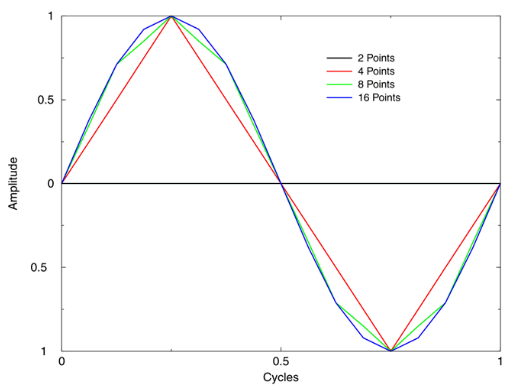5. Size of an element
• Typical variable name - box
• Calculated from the wavelength divided by number of elements per wavelength

```c frequency and meshsymb freqint =  1e6                  /* frequency of interest symb freqdamp =  \$freqint            /* set damping frequency to frequency of interestsymb vel =  1500                     /* minimum material velocity in model
symb wavelength =  \$vel / \$freqint   /* calculate smallest wavelength to disctretisesymb nepw =  15                      /* number of elements per wavelength symb box =  \$wavelength / \$nepw      /* calculate element size for meshing```

The freqdamp variable is not used as part of the meshing process but, it is usually set here with the value of freqint to align the damping characteristics of the materials over the correct frequency band of interest. This will be explained in a bit more detail in the next part of the tutorial.

Note: more elements per wavelength can be used to increase accuracy or minimise numerical dispersion*, however, this MUST be traded off against additional computational time. For example, in a 3D model, doubling the amount of elements per wavelength with multiply the model size by a factor of 8. Therefore, the effect of diminishing returns must be considered. Unless it is absolutely necessary, a small increase in accuracy is not necessarily worth the x10-20 increase in simulation time

*Numerical Dispersion is inherent in all numerical codes and is a small cumulative error that is caused due to sampling a wave in space and time. The non-physical phenomenon causes different frequencies to travel a different velocities; changing the shape of the wave with in turn can lead to amplitude (time domain) and phase (frequency domain) errors.

### #keycord:

Keypoints are critical points in space that will be used to accurately map out the dimensions of your geometry. Using the variables created in the Model Inputs section, we can use the #keycord command to generate these keypoints along the X and Y axis:

`c create x-y-z keypoints to accurately model dimensionssymb #keycord x 1 0.0 \$pzt_rad \$water_sizesymb #get { idx } rootmax x   /* store last keypoint indice in variable 'idx'            symb #keycord y 1 0.0 \$water_size \$pzt_thk \$water_sizesymb #get { jdx } rootmax y   /* store last keypoint indice in variable 'jdx'`

The #keycord command is very simple to set up:

1. Access the command through symb command
2. The first input argument is the direction which the keypoint will be generated: x,y or z (3D models)
3. The indice value which the keypoint will start from :1
4. The absolute value which the first defined keypoint
5. The dimensions to define folllowing keypoints: variables created

What the code will yield: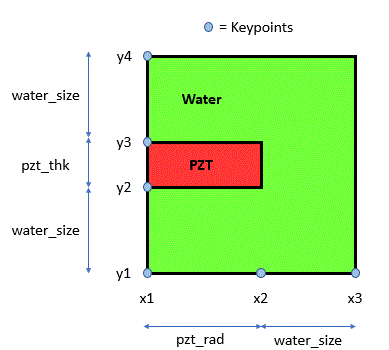Tip: we always record the last indice value using the #get rootmax option. Note that #get operations use braces { } instead of regular brackets ( )

It is useful to have quick access the last node/keypoint on the grid to use in additional commands and for debug purposes.

### #keyindx:

Following on from the XYZ keypoints, we need to determine how many elements are required to represent the distances along the XY direction which for the computatinal grid shall also be known as the IJ direction. We can use the dedicated symb command, #keyindx to do so:

`c create i-j-k keypoints symb #keyindx i 1 \$idx 1 \$box 1  /* calculate number of nodes required in i directionsymb #keyindx j 1 \$jdx 1 \$box 1  /* calculate number of nodes required in j direction          symb indgrd = \$i\$idx             /* last node number in i directionsymb jndgrd = \$j\$jdx             /* last node number in j direction`
1. Access the command through symb command
2. the first input argument is the direction which the keypoint will be generated - i, j or k(3D models)
3. the first keypoint indice value - 1
4. the last keypoint indice value - \$idx \$jdx or \$kdx
5. keypoint incremental value - 1
• This value allows keypoints to be skipped for the number of elements between keypoints calculation (i.e. 1 = (x1-x2-x3-x4-x5) or 2 = (x1-x3-x5) etc.)
6. maximum size of element - \$box
7. minimum spacing between keypoints - 1
• Places at least one element by default in between keypoints to prevent errors
• If value = 4, a minimum of 4 elements will always be created in between keypoints

Note: we recommend that element aspect ratio should not exceed 4:1 for stability and accuracy reasons so be careful when you are setting minimum spacing value to a higher integer value

### GRID and GEOM command:

`c create gridgrid \$indgrd \$jndgrd axiy     /* generate grid of nodes and apply axisymmetric condition to model gridc map physical geometry to grid of nodesgeom keypnt \$idx \$jdx        /* tie in all xy keypoints (physical dimensions) to ij keypoints(nodes)`

The grid command is very simple: it will generate a grid of evenly spaced nodes/elements based on the integer values input and allows the grid to be axially rotated to create axisymmetric models.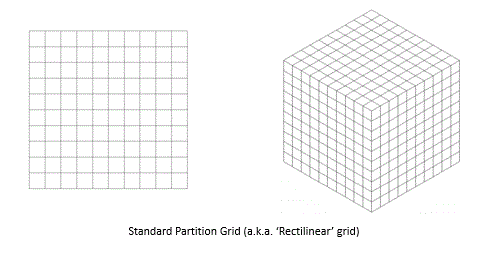The geom command ties in the physical distances to the nodal spacing, and where there is a keypoint, a node must lie at that precise location. This means that your mesh will look more like this: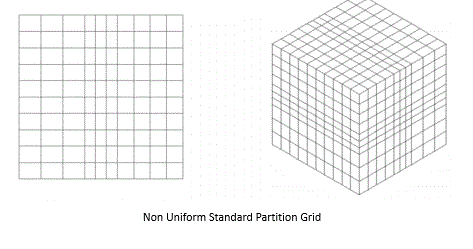This is one of the reasons why we set up models using keypoints: so that the important aspects of our geometry are at the exact required size.

### Meshing Section Code

`c frequency and meshsymb freqint =  1e6               /* frequency of interest symb freqdamp =  \$freqint         /* set damping frequency to frequency of interestsymb vel = 1500                   /* minimum material velocity in model symb wavelength = \$vel / \$freqint /* calculate smallest wavelength to disctretisesymb nepw = 15                    /* number of elements per wavelength symb box = \$wavelength / \$nepw    /* calculate element size for meshingc create x-y-z keypoints to accurately model dimensionssymb #keycord x 1 0.0 \$pzt_rad \$water_sizesymb #get { idx } rootmax x /* store last keypoint indice in variable 'idx' symb #keycord y 1 0.0 \$water_size \$pzt_thk \$water_sizesymb #get { jdx } rootmax y /* store last keypoint indice in variable 'jdx'c create i-j-k keypoints symb #keyindx i 1 \$idx 1 \$box 1 /* calculate number of nodes required in i directionsymb #keyindx j 1 \$jdx 1 \$box 1 /* calculate number of nodes required in j direction symb indgrd = \$i\$idx /* last node number in i directionsymb jndgrd = \$j\$jdx  /* last node number in j directionc create gridgrid \$indgrd \$jndgrd axiy /* generate grid of nodes and apply axisymmetric condition to model gridc map physical geometry to grid of nodesgeom keypnt \$idx \$jdx        /* tie in all xy keypoints (physical dimensions) to ij keypoints(nodes)`

## Materials

### Material Properties

The material types most commonly used in OnScale are:

#### Linear Elastic Isotropic (type elas)

• Material properties that are consistent in all axis directions
• Minimum required properties are density, bulk and shear moduli

Example Code:

```matr
type elas
wvsp off
prop epoxy \$density \$bulkmod \$shearmod  ```

Or using wave velocites:

```matr
type elas
wvsp off
prop epoxy \$density \$vellong \$velshear```

Tip: use the wvsp command to allow material to be defined by longitudinal and shear velocities

#### Anisotropic - Piezoelectric (type lean)

• Material properties are unique in each axis direction.
• Accurate modelling of piezoelectric materials requires the following parameters
• Density
• 6 stiffness [c] or compliance [s] components
• 3 piezo electric stress [e] or strain [d] constants
• 2 dielectric constant [εS]

Tip: By default OnScale accepts: constant stiffness parameters [c]; piezoelectric stress constants [e] and dielectric properties under constant strain [εS]

Example Code:

```matr
type lean
prop pmt3 \$rho
\$c11  \$c12  \$c13  0.0   0.0    0.0   \$c11
\$c13  0.0   0.0   0.0   \$c33   0.0   0.0
0.0   \$c44  0.0   0.0   \$c44   0.0   \$c66

piez pmt3 1 5 \$ex5 2 4 \$ex5 3 1 \$ez1 3 2 \$ez1 3 3 \$ez3
elec pmt3 \$epxx \$epxx \$epzz```

#### Nonlinear - Biological (type tisu)

• Nonlinear effects can be supported provided that pressure levels are high enough to initiate them.
• Additional material properties require additional inputs over conventional linear elastic materials:
• B/A - B over A (commonly know for biological materials)
• Maximum pressure cut-off - negative number as compression is negative in OnScale
• Minimum pressure cut-off - set to a large positive number for wave 'steepening'

Example Code:

`matr    wvsp on    type tisu    symb pmax = 2.e6    symb pmaxn = -2.e6    symb ba = 10.0    prop fatnl 928. 1427. 0. 0.01 0.0 0.0    \$ba \$pmaxn \$pmax`

#### Material Damping

Material damping must also be defined to accurately simulate your materials. OnScale offers a number of damping models to be used depending on your material type:

• Stiffness damping - sdmp command
• Mass damping - mdmp command
• Rayleigh damping - rdmp command
• Viscoelastic damping - vdmp command

The method of definition is similar across each of the damping models and requires the following information:

1. name of the material
2. frequency at which the damping model is centred at
3. damping option allows the convention of damping values to be set - Q-factor (q); dB/distance (db) or Fraction of critical damping (crit)
4. dilatational wave attenuation value
5. shear wave attenuation value
6. Frequency at which the attenuation values were measured at
7. exponent to control how damping varies with frequency
8. Only if option was set to db – sets the distance over which the damping values are achieved

Example 1:

`vdmp epoxy \$freqdamp db \$attlong \$attenshear \$measuref \$exponent \$dist `

Example 2:

`rdmp piezo \$freqdamp q \$attlong \$attenshear \$measuref \$exponent `

#### Material Database

OnScale provides an extensive material database to browse and select from using our new Material Database Tool. This allows you to create a custom project material file with all the code definitions, ready to be imported into your model file: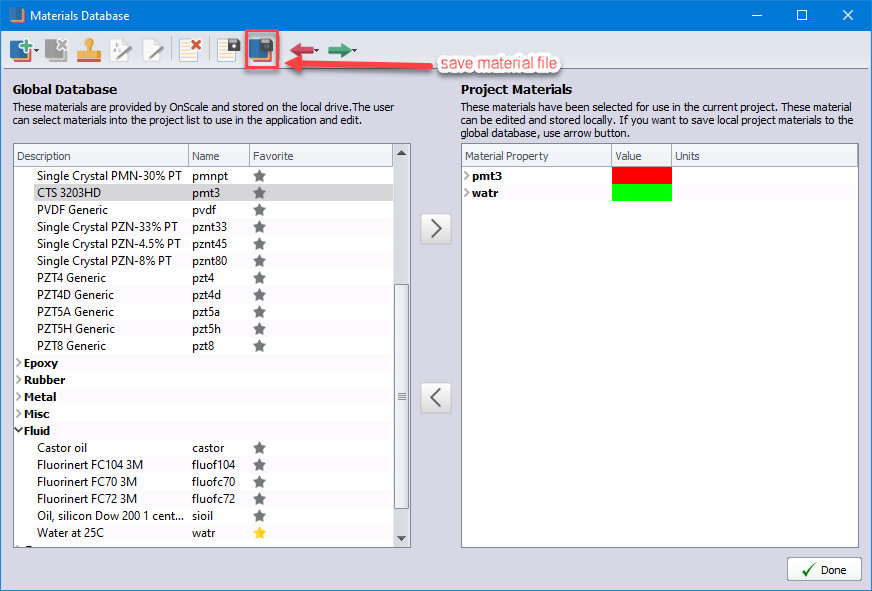Note you will need to modify the piezoelectric material (pmt3) poling direction to Y+ to match the thickness direction of the model.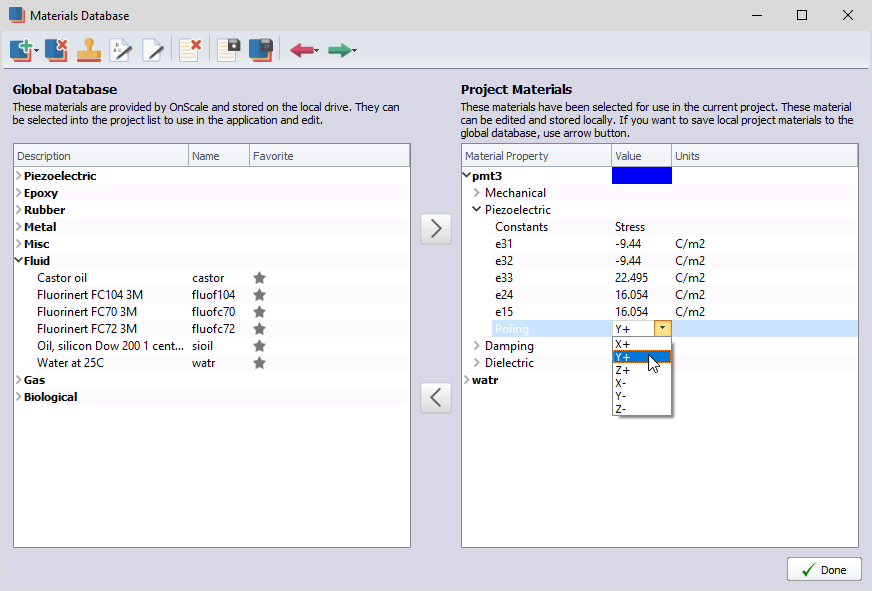```c material defintions
symb #read training.prjmat /* materials stored in .prjmat file and read into flex model ```

The OnScale material database are an excellent starting point if you do not have the exact properties for the materials you are using. Material properties tends to be the weakest link in the simulation chain due to the lack of information provided by the manufacturer and difference in properties from batch to batch.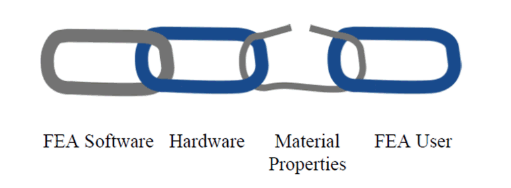Note: if possible, characterise the materials you are using for accurate simulation results otherwise, be aware that there will be slight discrepancies when compared to experimental results

### Assigning Materials to Grid

#### SITE:

The site command is what is used to apply materials to the model grid. There are a number of different primative shapes to allow you to recreate your desired geometry. The site command operates like paint brush a paint canvas, the last material application will appear on top and overwrite any material previously applied to the same region. Looking at our model geometry: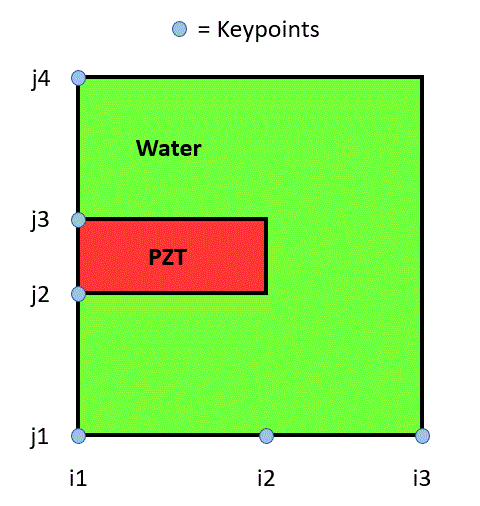The simplest method will be to apply water to the full model grid and the overwrite the smaller region with PZT using the regn command:

`c allocate materials to grid site      regn watr                 /* assign watr to full grid      regn pmt3 \$i1 \$i2 \$j2 \$j3 /* assign material 'pmt3' to grid defined by nodal range end `

Tip: the end command tells the compiler that it is no longer executing subcommands within a primary command. Although not required, it is good coding practice to include this to clearly signal the end of primary commands.

#### Checking the Model:

With the structure of the model set up, we should always check that we have applied materials to the correct part of the grid. For any plotting requirements, the grph command should be used to configure and plot any available model data:

```c plot model
grph
nvew 2 2     /* set up 2 views
plot matr    /* plot model
mirr x on    /* apply visual symmetry
plot matr    /* replot model
end
term             /* pause model ```
• nvew - controls the plotting window arrangement
• plot - plots any requested data array
• mirr - applies symmetry to the graphical plot
• term - pauses model to allow user input in the terminal

The FEA model at this point can be plotted by running the software for the first time. The compiler will execute all lines of code sequentially up until the it reaches the term command. Click on the Run icon to execute the model and the following Runtime Interactive Graphics window will appear: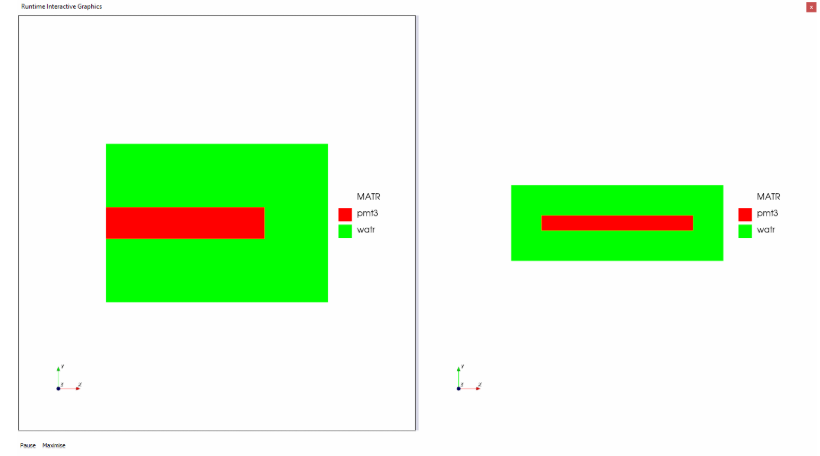### Material Section Code

`c material defintions symb #read training.prjmat /* materials stored in .prjmat file and read into flex model c allocate materials to grid site     regn watr /* assign watr to full grid     regn pmt3 \$i1 \$i2 \$j2 \$j3 /* assign material 'pmt3' to grid defined by nodal range end c plot model grph     nvew 2 2 /* set up 2 views     plot matr     /* plot model     mirr x on     /* apply visual symmetry     plot matr     /* replot model     end term      /* pause model `

### Boundaries of a Model

Now that we have fully assigned material to the entire grid, the external boundaries of the water need to be set up correctly to reflect how the areas beyond the model would behave in the real world. OnScale has a number of pre-defined boundary conditions to be applied by the user with the boun side command. The most commonly used boundary conditions are:

• free
• nodes can move freely with no external forces acting upon them
• When to use: edge of model is at
• symm
• boundary for symmetrical geomtries
• assumed that what occurs on one side occurs on the other
• When to use: model is the same geometrically and electrically across the boundary
• absr
• energy continues past boundary with no reflections
• When to use: edge of model continues infinitely with the same material (typically a large body of water)
• fixd
• nodes are completely fixed - zero displacement
• energy is perfectly reflected
• When to use: edge of model is infinitely stiff

The model should be set up with the following boundaries to simulate the PZT disc in a infinite body of water:

```c boundary conditions & loading

c external boundary conditions
boun
side xmin symm    /* assign symmetry condition xmin side of model
side xmax absr    /* assign absorbing boundary condition (infinite medium)
side ymin absr    /* to xmax ymin and ymax side
side ymax absr
end ```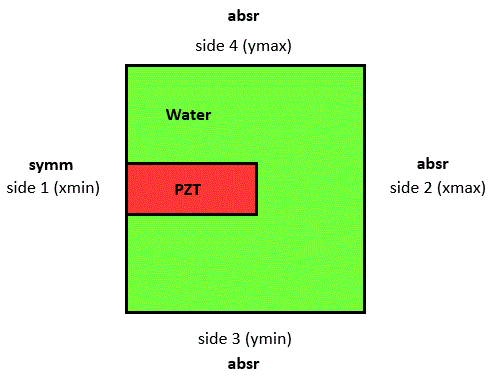### Time Functions & Piezoelectric Loads

The model has now been fully geometrically defined which means we can technically execute the model however, the model will remain static over time. We need to provide some form of input to stimulate the model which typically falls into 2 categories:

1. Pressure loads - for simulating purely mechanical wave problems
2. Piezoelectric loads - for simulating piezoelectric devices

Before we set up the loading conditions, an input waveform, also referred to as a Time Function, is required. OnScale provides a variety of Time Functions to use in the model ranging from sinusoids, wavelets and user-defined functions too. See func for a full list of input types. There is also a Time Function Tool to set up and visualise the input signals to be used within the model.

Tip: OnScale recommends using the Ricker Wavelet (wvlt) time function due to its broadband performance and low spectral leakage beyond 2.5x the centre frequency.

```c define time varying function
func wvlt \$freqint 1.	/* wavelet impulse function

symb ascale = 6.28	    /* area scaling for electrode area - required for accurate simulation of impedance amplitude - not required for axiy model

piez
wndo \$i1 \$i2 \$j2 \$j3    /* define electric window to pzt material - minimise for faster model run time

defn top        /* define electrode - name 'top'
node \$i1 \$i2 \$j3 \$j3    /* assign nodes for top electrode

defn bot         /* define electrode - name 'bot'
node \$i1 \$i2 \$j2 \$j2    /* assign nodes for bottom electrode

/* electrical boundary condition
bc top volt func        /* apply voltage condition to 'top' electrode and assign driving signal 'func'
bc bot grnd             /* apply ground condition to 'bot' electrode

slvr pard               /* use pardiso solver - our new faster electrostatic solver for piezo simulations
end ```

Defining the piezoelectric load requires the piez primary command and the following subcommands:

• wndo
• Define the electric window - the region of the grid desginated for the electro-mechanical calculation
• OnScale uses a hybrid method for the calculation by using an implicit (for the electrical calculations)and explicit solver (for the mechanical calculations) to minimise runtime.

Tip: Always try to minimise the electric window size to reduce model runtime and memory requirements.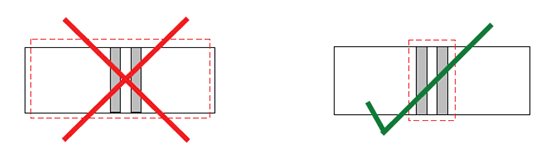• defn
• To initiate the definition of an electrode within the model with a reference name
• Also used to set the electrode area scaling to accurately simulate the capacitance of a piezoelectric device
• The simulated area must be same as the electrode area on your physical device
• For 2D models - must multiply by the dimension in the unmodelled direction and account for any symmetry conditions (x2 multiplier for each symm condition)
• For 2D axisymmetric models - no scaling is required as solver knows the exact size of device
• For 3D models - only need to account for symmetry conditions(x2 multiplier for each symm condition)
• node
• define the exact nodes on the grid to create the electrical boundary
• node command must follow the defn command
• bc
• applies electrical boundary conditions (ground, voltage, open etc...) to the electrodes generated by the defn and node commands
• slvr
• Selects the solver to use for the electrostatic calculations.
• pard is typically the fastest solver especially for large piezoelectric models

Tip: Alternative solvers will be slower however, some will offer a solution using much less memory if there are hardware limitations. See slvr.

`c boundary conditions & loading c external boundary conditionsboun    side xmin symm /* assign symmetry condition xmin side of model    side xmax absr /* assign absorbing boundary condition (infinite medium)    side ymin absr /* to xmax ymin and ymax side    side ymax absr     end c define time varying functionfunc wvlt \$freqint 1. /* wavelet impulse function c piezoelectric loadssymb ascale = 6.28 /* area scaling for electrode area - required for accurate simulation of impedance amplitude - '1' for axiy model piez    wndo \$i1 \$i2 \$j2 \$j3  /* define electric window to pzt material - minimise for faster model run time    defn top       /* define electrode - name 'top'    node \$i1 \$i2 \$j3 \$j3  /* assign nodes for top electrode     defn bot       /* define electrode - name 'bot'    node \$i1 \$i2 \$j2 \$j2  /* assign nodes for bottom electrode /* electrical boundary condition    bc top volt func      /* apply voltage condition to 'top' electrode and assign driving signal 'func'     bc bot grnd           /* apply ground condition to 'bot' electrode     slvr pard             /* use pardiso solver - our new faster electrostatic solver for piezo simulations     end `

## Model Outputs

### Calculated Properties

By default OnScale will calculate the velocity arrays for a simulation. Anything that is required beyond these arrays must be requested explicitly in order to be calculated and stored. The most common calculated data arrays are:

• Pressure: pres & aprs
• Displacement: disp (accessed through: xdsp, ydsp, zdsp)
• Stresses: strs (all 6 components accessed through the sg arrays e.g. sgxx sgyy...)
• Strain: strn (all 6 components accessed through ep arrays e.g. epxx epyy...)
• Loss: loss
• Maximum data arrays: max

For example, the code would look like:

```calc
pres aprs          /* calculate pressure and acoustic pressure
disp               /* calculate displacement
strn               /* calculate strain
strs               /* calculate stress
loss               /* calculate losses
max aprs pmin pmax /* capture minimum & maximum acoustic pressure and store in 'pmin' & 'pmax' array
end ```

For our PZT disc example, we require acoustic pressure, displacements and the maximum acoustic pressure field:

```calc
pres aprs          /* calculate pressure and acoustic pressure
disp               /* calculate displacement
max aprs none pmax /* capture maximum acoustic pressure only and store in 'pmax' array
end ```

### Output Types

#### Time Histories:

Time Histories in OnScale are simply data fields captured over time for specific nodes/elements in the model.

As a time-domain solver, OnScale can generate any of the calculated data arrays as a set of time histories. All that is required is to request the data at specific locations on the IJK grid which can be done using the pout and the following subcommands:

• hist
• Allows data field time histories to be extracted for nodes and elements on the IJK grid
• Example:
```pout
hist func                        /* time function - does not require ijk location
hist pres \$i1 \$i1 1 \$j3 \$j3 1    /* pressure for a single element
hist pres \$i1 \$i2 1 \$j3 \$j3 1    /* pressure for every elements in from i1 and i2
hist pres \$i1 \$i2 2 \$j3 \$j3 1    /* pressure for every 2nd element from i1 and i2
end ```
• histname
• Allows time histories to be extracted for specific named objects without the need to input IJK indice locations
• Example:
```pout
histname electrode vqi all    /* voltage, charge & current data for all electrodes defined
histname electrode vq top     /* voltage & charge data for 'top' electrode
histname avrg a all           /* store all requested averages
histname avrg a avepres       /* store the average with name 'avepres'
end ```

All time histories are given an array number in the sequence they are defined. The array numbers gives you access to the data array and can be used for plotting purposes if we want to visualise these signals during the model execution stage. This will be demonstrated later on in the tutorial. For our PZT example, we want to extract the time function, voltage and charge on all electrodes and the Y-displacement at the centre point on the top PZT surface:

```pout
hist func                         /* time function - array '1'
histname electrode vq all         /* voltage and charge data for all electrodes - array '2-5'
hist ydsp \$i1 \$i1 1 \$j3 \$j3 1     /* y-displacement at node on the top electrode - array '6'
end```

#### Mode Shapes:

Mode shapes are extracted through Harmonic Analysis methods. In OnScale, we can extract the harmonic behaviour of a system by performing DFTs at specified frequencies of interest. This can also be performed for specific data fields of interest, for example pressure, x-displacement and many others. It is a powerful tool as it can provide insights into system behaviour that may be difficult to achieve in a other ways.

The advantages of performing harmonic analysis in this manner, rather than with a modal solver, is that this method takes into account damping and mode-coupling in a more effective manner. It will therefore correspond more directly with experimental measurements from laser vibrometers taken in a similar manner i.e. driving a system at a constant frequency.

Mode shapes can be created via the shap command. This allows the user to specify the harmonic frequencies (freq) they are interested in along with any additional data fields (data) they wish to extract, for example:

```shap
data pres    /* pressure data to be extracted with mode shapes
data xdsp    /* x displacement data to be extracted with mode shapes
freq 1.5e6   /* mode shape '1'
freq 3.e6    /* mode shape '2'
end ```

For our PZT example, we want to extract the mode shape at the frequency of interest \$freqint along with the displacement data in the X and Y directions:

```shap
data xdsp       /* x displacement data to be extracted with mode shape
data ydsp       /* y displacement data to be extracted with mode shape
freq \$freqint   /* mode shape '1'
end ```

#### Extrapolation:

OnScale works most efficiently when models are on the order of tens of wavelengths in size along each axis. Medical and Sonar devices, however, commonly require predictions of pressure amplitudes at distances many hundreds of wavelengths from the source. These are either impractical or inefficient to model fully. The best approach in such cases is to extrapolate.

The Kirchoff extrapolation technique is a powerful tool incorporated into OnScale. It uses analytic techniques (essentially a combination of Green's functions and Huygen's principle) to take pressure/time data from the finite-element model and extrapolate to a full pressure/time response at any arbitrary location or locations.

The technique is valid in both near and far fields, but makes several assumptions:

1. Propagating medium is homogenous, i.e., no intervening layers
2. Propagating medium is fluid, i.e. no shear waves
3. Propagating medium is either lossless or very low loss
4. Response is linear

Tip: ideally, the extrapolation boundaries should encompass the full device in water but this is not always practical. In such cases, place the boundaries around as much of the device as possible. The boundary should be close but not be in contact with the device, typically 5 - 10 elements from the transducer: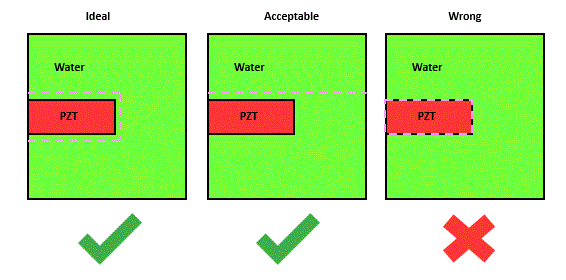For our PZT example, we will set up a single extrapolation boundary across the top of the device with a few lines of code:

```extr
defn kirc                        /* define type extrapolation boundary type - kirchoff
ref in \$x1 \$y2                   /* reference point to determine extrapolation vector
node \$i1 \$indgrd-1 \$j3+5 \$j3+5   /* extrapolation boundary at the defined nodal indices
driv func                        /* storing time function to allow tvr calculation in toolkit
end```

extr is the extrapolation primary command which contains the subcommands to set up the extrapolation calculation:

• defn
• To define the type of extrapolation boundary type to use - Kirchoff (kirc) is best suited option to use
• ref
• To determine which side of extrapolation boundaru to extrapolate out from
• The reference point can be anywhere in your model - you must correctly define whether the point is inside (in) or outside (out) the extrapolation boundary. Outside being the direction of the infinite medium where you are interested in the extrapolated outputs.
• node
• Defines the extrapolation boundary on the model grid using the IJK indices
• driv
• Stores the drive function in the extrapolation output file to allow for Transmit Voltage Response (TVR) calculation.

The analysis of the mentioned outputs will be discussed in more detail in the Post Processing section.

### Model Outputs Section Code

` calc      pres aprs          /* calculate pressure and acoustic pressure      disp               /* calculate displacement      max aprs none pmax /* capture maximum acoustic pressure only and store in 'pmax' array      end  pout      hist func                     /* time function - array '1'      histname electrode vq all     /* voltage and charge data for all electrodes - array '2-5'      hist ydsp \$i1 \$i1 1 \$j3 \$j3 1 /* y-displacement at node on the top electrode - array '6'      endshap     data xdsp      /* x displacement data to be extracted with mode shape     data ydsp      /* y displacement data to be extracted with mode shape     freq \$freqint  /* mode shape '1'     end extr     defn kirc                      /* define type extrapolation boundary type - kirchoff     ref in \$x1 \$y2                 /* reference point to determine extrapolation vector     node \$i1 \$indgrd-1 \$j3+5 \$j3+5 /* extrapolation boundary at the defined nodal indices     driv func                      /* storing time function to allow tvr calculation in toolkit     end`

## Process Model

### PRCS Command

Before running the model, you should always issue the prcs command. In preparation for processing the time-step information, it computes the model time step for stability and opens the necessary arrays for field-data storage.

`prcs`

Although the prcs command is automatically entered with the first exec command if it has not already been so, it is good practice to enter it before beginning. Once it is entered, no alterations to model geometry, material properties, and so on are permitted.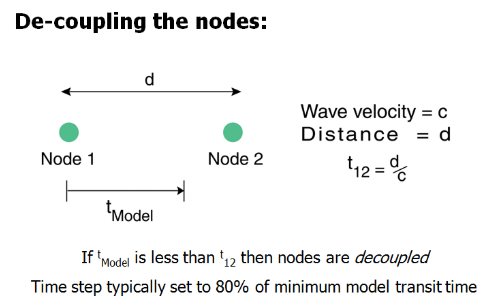Although 80% is default, it can be on the conservative side for some models. Users can adjust this upwards using the time command (must be issued before the prcs command) to help:

1. Reduce simulation time (larger timestep means less steps for a set simulation time)
2. Increase accuracy (reducing the amount of sampling in the time domain)

However, it is important to note than increasing it too much will eventually cause the model to become unstable, and care should be taken. A good value to try initially is 0.95:

`time * * 0.95 `

Increasing the TSF is also useful if you are running a model that propagates over longer distances (>25 wavelengths). By reducing the effect of sampling in the time domain, the solution at each timestep is more accurate, and therefore the cumulative effects of numerical dispersion can be lessened somewhat.

### Model Check

Typically after the prcs command, certain aspects of the model can be plotted and to check if they have been set correctly:

• model Boundaries
```grph
plot boun_type    /* plot model boundaries ```
• defined electrodes with poling arrows
```grph
arrow pole    /* turn on material poling arrows
plot piez     /* plot defined electrodes with poling arrows ```
• plot material geometry with electrodes and poling arrows
```grph
arrow pole        /* turn on material poling arrows
plot matr piez    /* plot defined electrodes over model geometry with poling arrows  ```
• plot material geometry with electrodes and poling arrows
```grph
arrow cstm 10 5 xvflow yvflow    /* set up custom vector arrows for flow velocities
plot xvflow yvflow               /* plot combination of x and y flow fields with velocity arrows  ```

For any piezoelectric model, it important to check if material poling has been set correctly otherwise, the device may not perform as expected. For most piezoelectric transducer applications, the poling should be in parallel with the thickness direction of the PZT. For our PZT example, this should be along the Y-axis. To change the poling direction of any material, we must access the it in our material file and locate the matr axis command for the PZT material we are using:

```...
elec pmt3 \$aepxx \$aepyy \$aepzz

piez pmt3 1 1 \$ex1 1 2 \$ex2 1 3 \$ex3 1 4 \$ex4 1 5 \$ex5 1 6 \$ex6 &
2 1 \$ey1 2 2 \$ey2 2 3 \$ey3 2 4 \$ey4 2 5 \$ey5 2 6 \$ey6 &
3 1 \$ez1 3 2 \$ez2 3 3 \$ez3 3 4 \$ez4 3 5 \$ez5 3 6 \$ez6

rdmp pmt3 \$freqdamp q \$qdmp \$qsdmp \$freqloss 1.0

axis pmt3 posy /* relate materials local system to global system
...  ```

Tip: by default, there are material axis definitions which can be used to control the material poling along each of the XYZ axes. Use the desired axis definition through its name: posx, negx, posy, negy, posz and negz.

For our PZT example we will set up the plotting with the following code:

```grph
nvew 2 1
line on	         /* turn on mesh lines
arrow pole          /* turn on poling arrows
plot piez	         /* plot created electrode
plot matr piez	 /* plot created electrode on top of model
end
term                     /* pause model to allow check```

### Process Model Section Code

```prcs     /* check models, sets up required data arrays, calculates stable timestep for solver to run model

grph     nvew 2 1     line on             /* turn on mesh lines     arrow pole          /* turn on poling arrows     plot piez           /* plot created electrode     plot matr piez      /* plot created electrode on top of model     end term                /* pause model to allow check```

## Model Execution

### Execution Setup & Running the Model

Following on from processing the model and final checks, we can run the model in a number of ways.

#### Basic Model Execution:

The basic information required prior to executing the model:

• Model timestep (step)
• Simulation runtime (simtime)
• Simulation runtime in terms of number of timesteps (nexec)
```c runtime calculations
symb #get { step } timestep    	/* extract calculated timestep from prcs command
symb simtime = 40 / \$freqint   	/* simulation time - 40 cycles @ 1 mhz
symb nexec = \$simtime / \$step  	/* determine how many timesteps to run full model```

Once we have the total number of timesteps to run the simulation for, we can use the exec command to execute the model. Typically, we would set up plots for to visualise the simulated outputs too:

```c running the model
exec \$nexec    	/* execute model for 'nexec' timesteps

c plot outputs
grph
nvew 3 1      /* set up 3 views for data plots
plot ydsp     /* plot calculated data array
plot pmax     /* plot max pressure data array
plot 2        /* plot time history 2 - pressure signal at front of defect
end
term    /* pause model to view results
```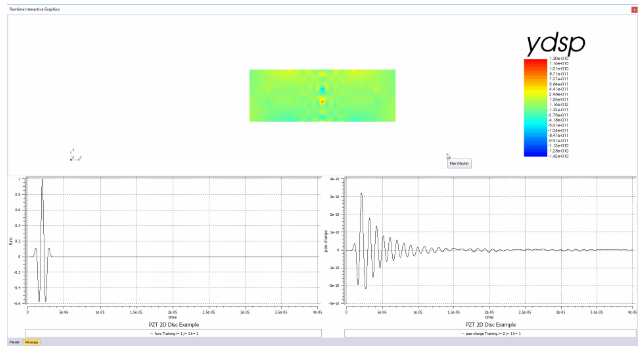Great! We ran our first simulation however, we may want to visualise data at points thoughout the model simulation for analysis/debugging purposes. We can improve on this method by using a do loop.

#### Improved Model Execution:

Additional variables are required for the do loop method of executing the model:

• Number of do loop iterations(nloops)
• Number of timestep executions per do loop iteration (nexec2)
```c runtime calculations
symb #get { step } timestep    	/* extract calculated timestep from prcs command
symb simtime = 40 / \$freqint   	/* simulation time - 40 cycles @ 1 mhz
symb nexec = \$simtime / \$step  	/* determine how many timesteps to run full model
symb nloops = 80               	/* plotting snapshots during model execution
symb nexec2 = \$nexec / \$nloops 	/* partition full simulation into smaller segments for plotting```

The do loop will have to loop nloops times, and will have to execute the model for nexec2 timesteps before plotting the results to the interactive graphics window.

```c setting up output plots
grph
arrow off  /* turn off arrows
line off   /* turn off mesh lines
nvew 3 1   /* set up 3 plotting windows in layout number 1
end

do loopi i 1 \$nloops 1  /* setting up do loop
exec \$nexec2    	/* run model for 'nexec2' timesteps

grph
plot ydsp       /* plot calculated data array
plot 1    	/* plot time history 1 - drive function
plot 3    	/* plot time history 3 - charge on top electrode
imag      	/* snapshot of graphics window for movie generation
end
end\$ loopi
term                    /* pause model to view results```

The do loop is very simple to set up:

1. Initiate the do command
2. Assign the do loop with a name(loopi)
3. Assign the do loop with a loop variable (i) i.e. to control how many loops to run for
4. Define start and end value the loop variable(1 to \$nloops)
5. Define incremental value to step through from start to end value(1)

Place the exec and grph commands within the do loop and terminate the do loop using the end\$ command followed by the name of the loop.

Tip: Always minimise the amount of execution code within a do loop or procedure for optimal runtime performance. Avoid placing grph setup commands within the execution loops such as nvew, mirr etc.

The do loop has allowed us to view the simulated results at interval stages of the model right through until the end of the simulation however, there may be situations where we haven't simulated the model for a long enough time period. In such cases, we would need to restart the model and increase the simtime variable and re-run the entire model again which is very inefficient. We can further improve the model execution process using a procedure.

#### Optimal Model Execution:

A procedure is a segment of code that can be recalled a number of times using the proc command. It is almost identical to how we have set up the do loop; we simply replace the do loop with the proc setup commands:

```proc plot save     /* setting up procedure with name 'plot
exec \$nexec2       /* run model for 'nexec2' timesteps

grph
plot ydsp      /* plot calculated data array
plot 1    	   /* plot time history 1 - drive function
plot 3    	   /* plot time history 3 - charge on top electrode
imag      	   /* snapshot of graphics window for movie generation
end
end\$ proc          /* end procedure

proc plot \$nloops  /* call 'plot' procedure 'nloops' times
term               /* pause model to view results
```

So when the simulation reaches the term command now, the user can enter in the console input:

`p> proc plot 10`

which will execute procedure for an additional 10 times.

Tip: Always use a procedure to execute a model for greater control of your execution stage, should you ever need it.

### Extracting Outputs

After the simulation has fully executed, we can ask the solver to output certain data calculated during the simulation to an output file (.flxdato) ready to be post-processed. Most commonly we extract: the model geometry, any of the calculated properties (pres aprs xdsp loss pmax etc.) and any requested mode shape data.

```data
out modl        /* outputs model geometry
out pres        /* outputs pressure data field at the final simulated timestep
out shap/all    /* output all requested mode shape data
end ```

It is also good modelling practice to output all the symbol variables used in the model. This gives the user insight into settings used or perhaps to allow the variables to be read into a post processing script:

```c save symbols to file for later use
symb #get { label } jobname     /* get model job name
symb #save \$label.symb        	/* save symbol file

c end of input file
stop
```

Finally, issue the stop command to exit the system process in an orderly fashion.

### Model Execution Section Code

```c runtime calculations
symb #get { step } timestep    	/* extract calculated timestep from prcs command
symb simtime = 40 / \$freqint   	/* simulation time - 40 cycles @ 1 mhz
symb nexec = \$simtime / \$step  	/* determine how many timesteps to run full model
symb nloops = 40               	/* plotting snapshots during model execution
symb nexec2 = \$nexec / \$nloops 	/* partition full simulation into smaller segments for plotting
c setting up output plots
grph
arrow off     /* turn off arrows
line off      /* turn off mesh lines   set imag avi  /* set image capture for movie file generation
nvew 3 1      /* set up 3 plotting windows in layout number 1
end
c create procedure - code that can be executed 'x' number of timesproc plot save   /* name procedure 'plot' and save the codeexec \$nexec2     /* run model partially  grph    plot ydsp    /* plot calculated data array    plot 1       /* plot time history 1 - drive function    plot 3       /* plot time history 3 - charge on top electrode    imag         /* snapshot of graphics window for movie generation    end end\$ proc       /* end of procedurec run model by calling 'plot' procedure 'nloops' timesproc plot \$nloopsterm             /* pause model to view results data    out modl      /* outputs model geometry    out pres      /* outputs pressure data field at the final simulated timestep    out shap/all  /* output all requested mode shape data     end c save symbols to file for later use symb #get { label } jobname     /* get model job name symb #save '\$label.symb'        /* save symbol file c end of input file stop```

## Summary

All your models should follow the structure outlined in this tutorial example or be very similar in order to cover all aspects of your model setup. You will be able re-use the code from this model and develop it into models for your own applications. Here are is a checklist of questions you should always consider when building your model:

• Have all useful dimensions been captured to map out your geometry with keypoints?
• Has the mesh/grid been set up to accurately model your application?
• Have all the materials, to be used in the model, been defined and accessible in the file?
• Have materials been assigned to the correct location on the grid to recreate the desired geometry?
• Has the model been set up to interact with the external world beyond the model boundaries?
• Have all desired outputs been requested?
• Final checks before model execution?
• Run the model

• Always start with a small and simple 2D model - these are quick to run and can be used to debug set up issues, test new designs and confirm fundamental theories
• Comment throughout your model - helps others understand what is happening in your code as well as reminding yourself
• Keep your code tidy - much easier to spot coding errors when the code is structured well
• Used the debug window to figure out model issues - the debug show where errors encounter in your model file to help you pinpoint the issue
• Variables I J K L M N are integer variables by default - this is a very common error
• Use the command reference to figure out how to set up commands

### Analyzing Model Outputs

Within your model folder directory, output files will have been generated from the simulations which can include the following file types:

• Flex History File - .flxhst - stores all data requested by the pout command
• Flex Data Out File - .flxdato - stores any data field requested using the data out command
• Flex Extrapolation - .flxext - the extrapolation file generated from the extr command
• Flex Print File - .flxprt - The print file contains a log of all the lines of code executed with additional model data
• Flex Symbol File - .symb - contains all variables used in the model

You are now ready to analyse your model outputs using the Post Processor!!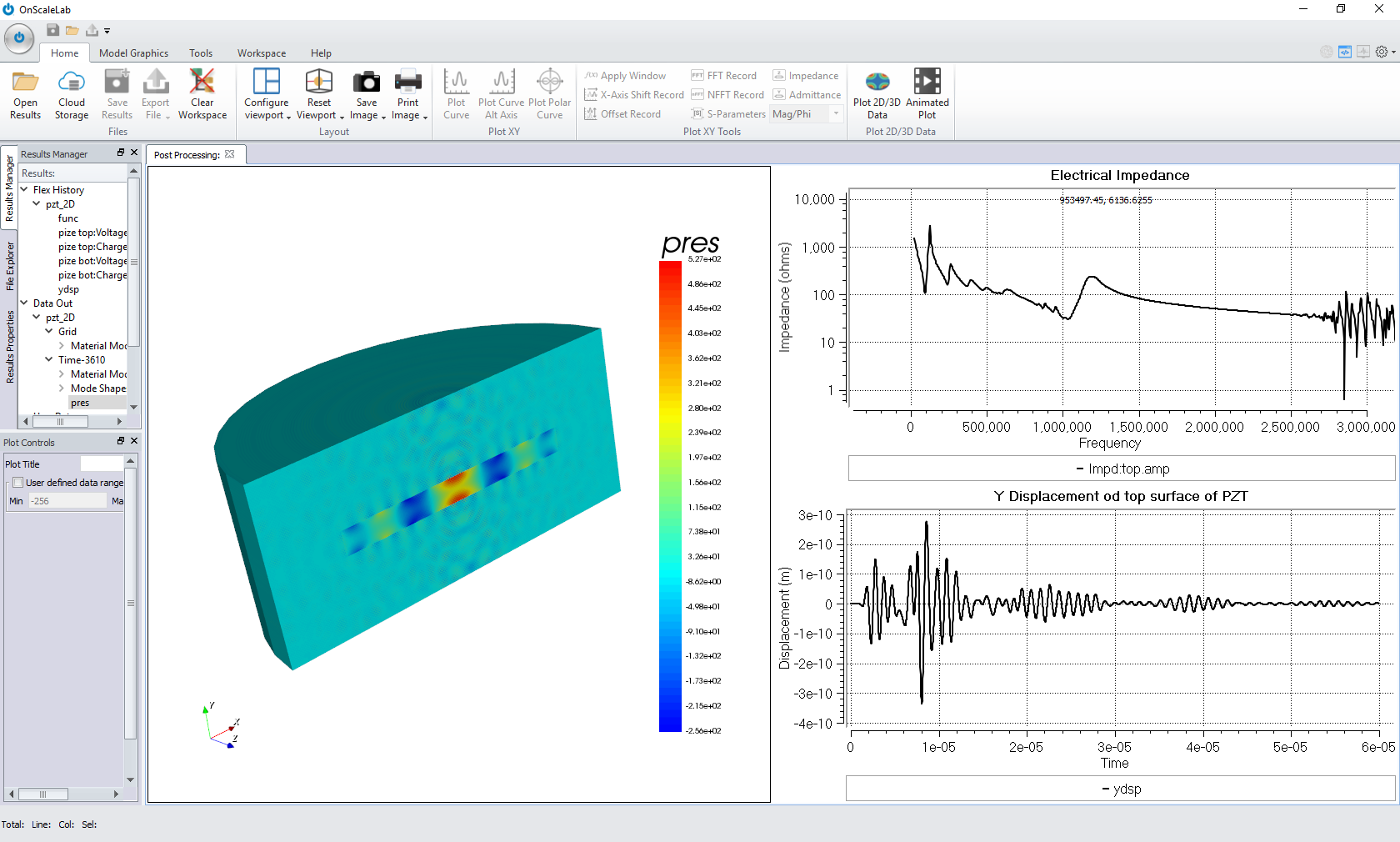## Running Parametric Sweeps

Any symb variable in the script can be switched to a parametric variable (symbx) that will allow you to sweep that particular parameter.

For example:

`symbx water_size = 4e-3 /* dimension of water around transducer symbx pzt_rad = 10e-3  /* pzt radiussymbx pzt_thk = 2e-3  /* pzt thickness`

To find out how to sweep these parameters on the cloud head to the following article: Cloud Workflow